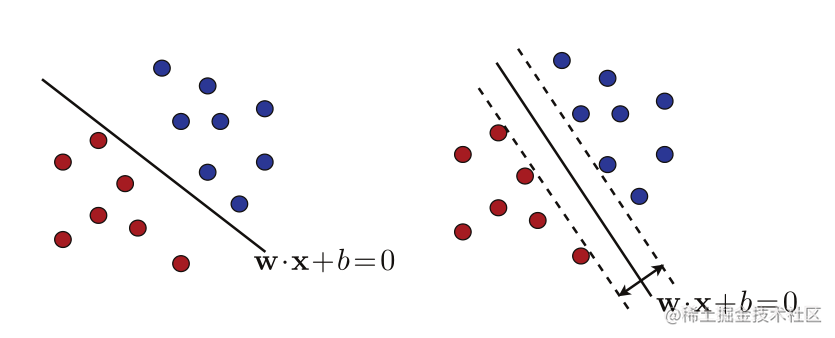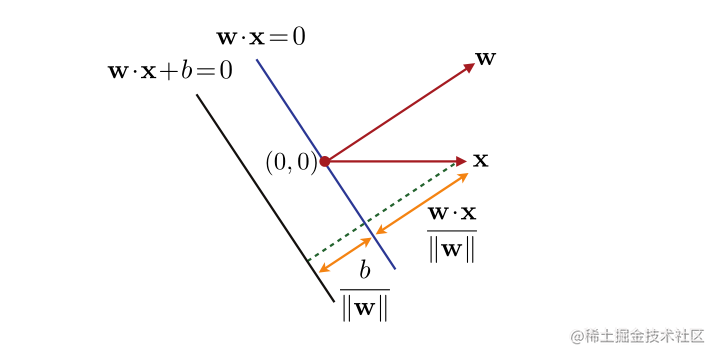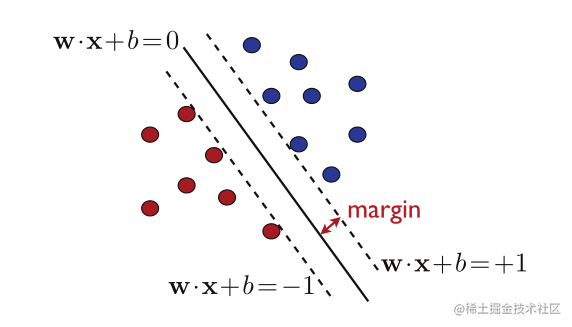# 5 支持向量机

## 5.1线性分类

$R_{\mathcal D}(h)=\underset {x\sim\mathcal D}{\mathbb P}[h(x)\neq f(x)].(5.1)$

$\mathcal H =\{x\mapsto sign(\bf w\cdot x+\Bbb b ):w\in\mathbb R^N,\Bbb b\in\mathbb R\}.(5,2)$## 5.2可分离情况

$\forall i\in[m],y_i(\bf w\cdot x_i+\Bbb b)\ge 0.(5.3)$

### 定义5.1（几何边界）

$p_h(x)=\frac{|\bf w\cdot x+\Bbb b|}{||\bf w||_2}.(5.4)$

SVM解是具有最大几何余量的分离超平面，因此称为最大余量超平面。图5.1的右面板显示了SVM返回的最大边距超平面可分离情况下的算法。我们将在本章后面介绍一种理论，为这种解决方案提供有力的理由。然而，我们已经可以观察到，SVM解决方案也可以被视为以下意义上的“最安全”选择：测试点通过具有几何边界ρ的分离超平面正确分类，即使它位于共享相同标签的训练样本的距离 $ρ$ 之内；对于SVM解决方案， $ρ$ 是最大几何裕度，因此是“最安全”的值。#### 图5.2

$\bf w·x>0$ $\Bbb b>0$ 的情况下，点 $\bf x$ 的几何边界的图示。

### 5.2.1原始优化问题

$p=\underset {\bf w,\Bbb b:\Bbb y_{\Bbb i}(\bf w\cdot x_{\Bbb i}+\Bbb b)\ge 0}{max}\underset {i\in[m]}{min}\frac{|\bf w\cdot x_{\Bbb i}+\Bbb b|}{||\bf w||}=\underset {\bf w,\Bbb b}{max}\underset {i\in[m]}{min}\frac{y_i(\bf w\cdot x_{\Bbb i}+\Bbb b)}{||\bf w||}.(5.5)$

$p=\underset {\underset{min_{i\in [m]y_i(\bf w\cdot x_{\Bbb i}+\Bbb b)=1}}{\bf w,b:}}{max}\frac{1}{||\bf w||}=\underset {\underset {\forall i\in [m],y_i(\bf w\cdot x_{\Bbb i}+\Bbb b)\ge 1}{\bf w,b:}}{max}\frac{1}{||\bf w||}.(5.6)$#### 图5.3

(5.6) 的最大边界超平面解。图上的虚线表示边缘超平面。

\begin{aligned} &\underset {\bf w, \Bbb b}{min}\frac{1}{2}||\bf w||^2\\ &受y_i(w\cdot x+\Bbb b)\ge \Bbb1,\forall \Bbb i\in [\Bbb m].的影响。(5.7) \end{aligned}

### 5.2.2支持向量

$\mathcal L(\bf w,b,\alpha)=\frac{1}{2}||\bf w||^2-\sum^m_{\Bbb i=1}\alpha_{\Bbb i}[\Bbb y_\Bbb i(w\cdot x+\Bbb b)-\Bbb 1].(5.8)$

\begin{aligned} \nabla_{\bf w}\mathcal L={\bf w}-\sum^m_{i=1}\alpha_iy_i\bf x_\Bbb i=0 \qquad&\Longrightarrow\qquad {\bf w} =\Bbb {\sum^m_{i=1}\alpha_i\Bbb y_i\bf x_\Bbb i}(5.9)\\ \nabla_{b}\mathcal L=-\sum^m_{i=1}\alpha_iy_i=0\qquad&\Longrightarrow\qquad \sum^m_{i=1}\alpha_iy_i=0(5.10)\\ \forall i,\alpha_i[y_i(\bf w\cdot x +\Bbb b)-\Bbb 1]=0\qquad&\Longrightarrow\qquad\alpha_i=0y_i(\bf {w\cdot x} +\Bbb b)-\Bbb 1).(5.11) \end{aligned}